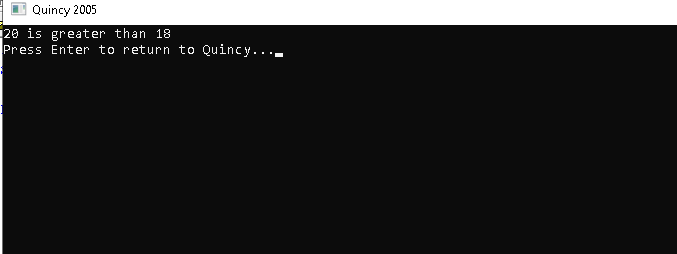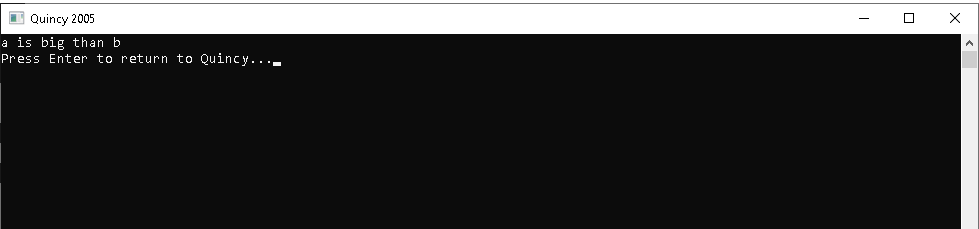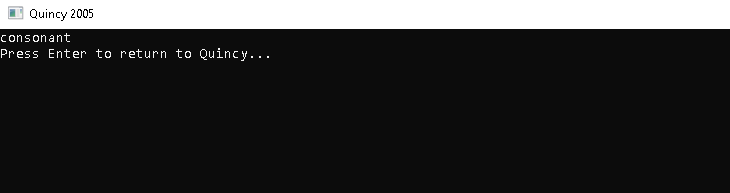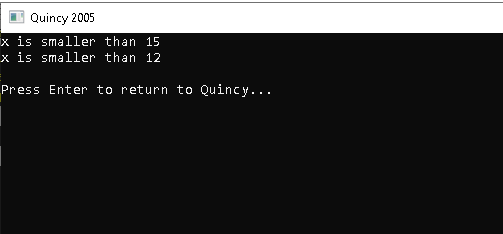# Decision statements

#### Decision statements

Decision-making structures require the program, together with a statement or statements that must be executed, that one or more conditions be assessed or tested in the program where the condition is adequately established, and optionally other statements that must be made if the state is found to be false.

#### The following types of decisions can be found in the programming language of C++.

Sr.No Statement & Description
1 if statement
It comprises of a boolean expression that is followed by some statements.
2 if...else statement
It can be followed by an ‘else’ statement, that executes when the expression of Boolean is false
3 switch statement
It allows a variable for testing the equality against some values.
4 nested if statements
You get to use an if-else statement inside another if or else-if
5 nested switch statements
You get to use a ‘switch’ statement inside another ones.

#### The ? : Operator

Exp1 ? Exp2 : Exp3;

There are expressions Exp1, Exp2, and Exp3. Please note that the colon is used and placed. This determines the value of a?' expression: Exp1 is assessed. Exp2 will be valued if trustworthy, and the whole '?' expression will be valued. If Exp1 is false, Exp3 will be evaluated, and its value will be the expression value.

#### if Statement

It comprises of a boolean expression that is followed by some statements.

#### Syntax

if (condition) { // block of code to be executed if the condition is true }

#### Example:

#include <iostream> using namespace std; int main() { if (25 > 8) { cout << "25 is greater than 8"; } return 0; }

#### OUTPUT:#### if...else statement

It can be followed by an ‘else’ statement, that executes when the expression of Boolean is false

#### Syntax

if (condition) { // block of code to be executed if the condition is true } else{ // block of code to be executed if the condition is false }

#### Example:

#include <iostream> using namespace std; int main() { int a=100,b=18; if (a > b) { cout << "a is big than b"; } else cout << "a is small than b"; return 0; }

#### OUTPUT:#### switch statement

It allows a variable for testing the equality against some values.

#### Syntax

switch(expression) { case x: // code block break; case y: // code block break; default: // code block }

#### Example

#include <iostream> using namespace std; int main() { char alph ='d'; switch (alph) { case 'a': cout << "vovel"; break; case 'e': cout << "vovel"; break; case 'i': cout << "vovel"; break; case 'o': cout << "vovel"; break; case 'u': cout << "vovel"; break; default: cout << "consonant"; break; } return 0; }

#### OUTPUT:#### nested if statements

You get to use an if-else statement inside another if or else-if

#### Syntax

if (condition1) { //condition1 is true then Executes if (condition2) { //condition2 is true then Executes } }

#### Nested-if statement

#include <iostream> using namespace std; int main() { int x = 10; if (x == 10) { // if statement true if (x < 15) cout <<"x is smaller than 15\n"; // only executed if statement above is true if (x < 12) cout <<"x is smaller than 12 \n"; else cout <<"x is greater than 15"; } else { cout <<"x is not equal 10"; } return 0; }

#### OUTPUT: